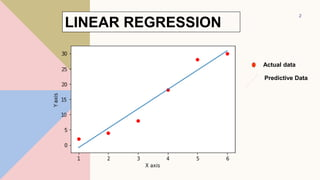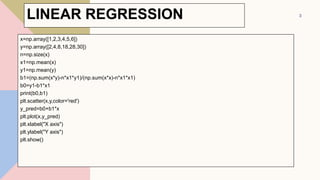Successfully reported this slideshow.

# Linear Logistic regession_Practical.pptx×

# Linear Logistic regession_Practical.pptx

Linear regression analysis is used to predict the value of a variable based on the value of another variable.

Linear regression analysis is used to predict the value of a variable based on the value of another variable.

### Linear Logistic regession_Practical.pptx

1. 1. LINEAR / LOGISTIC REGRESSION Dr. Amanpreet Kaur​ Associate Professor, Chitkara University, Punjab
2. 2. LINEAR REGRESSION 2 Actual data Predictive Data
3. 3. x=np.array([1,2,3,4,5,6]) y=np.array([2,4,8,18,28,30]) n=np.size(x) x1=np.mean(x) y1=np.mean(y) b1=(np.sum(x*y)-n*x1*y1)/(np.sum(x*x)-n*x1*x1) b0=y1-b1*x1 print(b0,b1) plt.scatter(x,y,color='red') y_pred=b0+b1*x plt.plot(x,y_pred) plt.xlabel("X axis") plt.ylabel("Y axis") plt.show() 3 LINEAR REGRESSION
4. 4. Write a python code to read (advertisement.csv) csv file to generate a plot with actual data (Red dots) and predictive data( blue line) with the help of linear regression and find out the Money spent on particular item as well sales of it. 4 LINEAR REGRESSION - EXAMPLE
5. 5. ADVERTISEMENT DATA( IN CSV FORMAT) unnamed:0 TV Radio Newspaper Sales 1 230.1 37.8 66.4 22.1 2 44.5 22.9 33.4 10.4 3 45.9 69.6 22.9 9.3 4 41.5 55 11 18 5 10.8 66.5 22.3 22 6 12.9 44.6 26 12.3 7 22.6 45.6 44.3 21.3 8 33.2 46.6 42.3 13 9 34.5 47.6 55.6 11 10 75.5 68.6 56.6 10 11 36.5 99.6 87.6 22 12 37.5 50.6 58.6 14.5 13 38.5 51.6 59.6 23 14 39.5 62.6 90.6 21 15 90.5 53.6 61.6 2.6 16 41.5 84.6 62.6 11 17 42.5 55.6 43.8 12 18 43.5 56.6 64.6 11.6 19 84.5 97.6 65.6 12.5 20 45.5 58.6 96.4 13.4 5
6. 6. import pandas as pd import numpy as np import matplotlib.pyplot as plt from sklearn.linear_model import LinearRegression from sklearn.metrics import r2_score import statsmodels.api as sm data = pd.read_csv("advertisement.csv") data.head() 6 LINEAR REGRESSION - EXAMPLE
7. 7. 7 sklearn.metrics.r2_score(y_true, y_pred, *, sample_weight=None, multioutput='uniform_averag • R^2 (coefficient of determination) regression score function. • Best possible score is 1.0 and it can be negative (because the model can be arbitrarily worse). • A constant model that always predicts the expected value of y, • disregarding the input features, would get a R^2 score of 0.0. LINEAR REGRESSION - EXAMPLE
8. 8. 8 LINEAR REGRESSION - EXAMPLE
9. 9. plt.figure(figsize=(10, 8)) plt.scatter( • data['TV'], • data['Sales'], • c='red' ) plt.xlabel("Money spent on TV ads (\$)") plt.ylabel("Sales (\$)") plt.show() 9 LINEAR REGRESSION - EXAMPLE
10. 10. 10 LINEAR REGRESSION - EXAMPLE
11. 11. predictions = reg.predict(X) plt.figure(figsize=(16, 8)) plt.scatter( data['TV'], data['Sales'], c='black' ) plt.plot( data['TV'], predictions, c='blue', linewidth=2 ) 11 LINEAR REGRESSION - EXAMPLE
12. 12. SIGMOID FUNCTION import math def sigmoid(x): a = [] for item in x: a.append(1/(1+math.exp(-item))) return a import matplotlib.pyplot as plt import numpy as np x = np.arange(-10, 10, 0.2) sig = sigmoid(x) plt.plot(x,sig) plt.show() 12
13. 13. LOGISTIC REGRESSION-EXAMPLE • Write down a python code for Logistic Regression where needs to read csv file(banking.csv) • Generate a bar graph after read the predictive variable Y • Calculate the percentage of Subscription / No Subscription. 13
14. 14. import pandas as pd import numpy as np from sklearn import preprocessing import matplotlib.pyplot as plt plt.rc("font", size=14) from sklearn.linear_model import LogisticRegression from sklearn.model_selection import train_test_split import seaborn as sns sns.set(style="white") sns.set(style="whitegrid", color_codes=True) 14 LOGISTIC REGRESSION-EXAMPLE